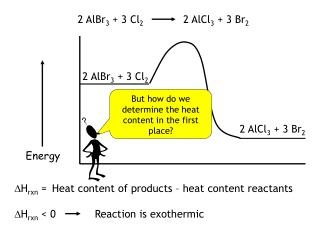DownloadDownload Presentation2 AlBr 3 + 3 Cl 2

# 2 AlBr 3 + 3 Cl 2

Télécharger la présentation## 2 AlBr 3 + 3 Cl 2

- - - - - - - - - - - - - - - - - - - - - - - - - - - E N D - - - - - - - - - - - - - - - - - - - - - - - - - - -
##### Presentation Transcript

1. 2 AlBr3 + 3 Cl2 2 AlCl3 + 3 Br2 2 AlBr3 + 3 Cl2 2 AlCl3 + 3 Br2 Energy But how do we determine the heat content in the first place? DHrxn = Heat content of products – heat content reactants DHrxn < 0 Reaction is exothermic

2. 2 AlBr3 2 Al + 3 Br2 DHrxn DHf(AlBr3) = 2 Heat of formation, DHf • The DHf of all elements in their standard state equals zero. • The DHf of all compounds is the molar heat of reaction for synthesis of the compound from its elements DHf (AlBr3): DHrxn = 2DHf(AlBr3) • Since the DHrxn can be used to find DHf, this means that DHf can be used to find DHrxnWITHOUT having to do all of the calorimetric measurements ourselves!! The Law of Conservation of Energy strikes again!!

3. Hess’s Law: DHrxn = S DHf(products) – S DHf(reactants) 6 CO2 (g) + 6 H2O (l) C6H12O6(s) + 6 O2(g) From DHf tables: DHf(C6H12O6) = -1250 kJ/mol DHf(CO2) = -393.5 kJ/mol DHf(H2O) = -285.8 kJ/mol DHrxn = [DHf(C6H12O6) + 6 DHf(O2)] – [6 DHf(CO2) + 6 DHf(H2O)] DHrxn = [-1250 kJ/mol] – [6(-393.5 kJ/mol) + 6(-285.8 kJ/mol)] DHrxn = +2825.8 kJ/mol

4. Using Hess’ Law with DHrxn What is the DHcomb for ethane? Target rxn: 2 C2H6 (g) + 7 O2 (g) → 4 CO2 (g) + 6 H2O (g) Given: DHrxn Rxn 1: -1323 kJ C2H4 (g) + 3 O2 (g) → 2 CO2 (g) + 2 H2O (g) Rxn 2: -137 kJ C2H4 (g) + H2 (g) → C2H6 (g) Rxn 3: 2 H2 (g) + O2 (g) → 2 H2O (g) -242 kJ 1) Rxn 1 doesn’t have enough oxygens, so multiply by 2 2(C2H4 (g) + 3 O2 (g) → 2 CO2 (g) + 2 H2O (g)) 2(-1323 kJ) 2) Rxn 2 is going the wrong direction and doesn’t have enough C6H6. Reverse reaction and multiply by 2. 2(+137 kJ) 2(C2H6 (g) → C2H4 (g) + H2 (g)) Note: when you reverse a reaction, change the sign on the H

5. 3) Combine the two reaction equations: Target rxn: 2 C6H6 (g) + 7 O2 (g) → 4 CO2 (g) + 6 H2O (g) 2 C2H4 + 6 O2→ 4 CO2 + 4 H2O 2(-1323 kJ) + 2(+137 kJ) + 2 C2H6 → 2 C2H4 + 2 H2 2 C2H4 + 2 C2H6+ 6 O2 → 4 CO2 + 4 H2O + 2 C2H4 + 2 H2 + 2 H2 + O2 → 2 H2O + (-242 kJ) 2 C2H6 + 2 H2+ O2 + 6 O2 → 4 CO2 + 2 H2O + 4 H2O + + 2 H2 4) Still don’t have enough O2 and need to get rid of H2. Add in Rxn 3. Final rxn: 2 C2H6 + 7 O2→ 4 CO2 + 6 H2O Math: 2(-1323 kJ) + 2(+137) + (-242) = -2614 kJ = Hcomb